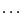Next: Troubleshooting: ALIAS does not Up: Examples of applications of Previous: Robot synthesis example   Contents

A quantum mechanics example

The Kochen-Specker theorem is one of the more important in quantum mechanics. Its a application involves the solving of the following problem (that has been simplified for the sake of clarity).

Considerunit vectors named 1,2,..9,A,B,..Z in a four dimensional space. A group of vectors is a set of 4 vectors that have to be orthogonal to each other and not identical or opposite. A m-sequence is a set of m groups such that:

• each vector in the sequence has the color black or white
• in each group of the sequence there must be exactly 3 black vectors and one white vector
The smallest known sequence is a 9-sequence named the Cabello's system:

1234,4567,789A,ABCD,DEFG,GHI1,35CE,29BI,68FH
which involves 18 vectors.

Our objective is to to make an exhaustive search of m-sequences. For that purpose we use a software designed by B.D. McKay, called the generator, that provides for any set of a vectors and any sequence with b groups all the b-sequences that satisfy the color constraints and are not isomorphic (for example changing the name of the vectors will lead to the same sequence). Then we consider each potential b-sequence and we must check if there are at least one set of coordinates for the vectors such that the orthogonality constraints for each group are satisfied together with the non-collinearity between the vectors.

Such verification is not so easy. Indeed the orthogonality constraints for a group involves 6 equations: consequently for a b-sequence with a vectors we have 6b orthogonality equations, a equations that indicate that the vectors are unitary. If we consider for example the Cabello's system the number of equations is therefore already 74 for 72 unknowns. Although the problem is algebraic the solving method in algebraic geometry have difficulties to handle such number of equations (for a sequence that is one of the smallest).

A second problem is that an exhaustive enumeration of all potential b-sequences leads to a very large number of sequences. For example for a=10 and b=12 the number of sequences is 197 885 058Nevertheless the problem was convenient for interval analysis. Indeed the unknown coordinates are component of unitary vectors and hence will lie in the range [-1,1]. Furthermore if a vector is solution then its opposite is also solution: hence we may restrict one of the coordinates of the vector to lie in the range [0,1]. In the same manner any rotation applied simultaneously to all solution vectors will lead to another set of solution vectors. Hence we may choose arbitrary any group so that its vectors constitutes an orthogonal basis of the four dimensional space. The coordinates of the vectors in this group will then be (1,0,0,0), (0,1,0,0), (0,0,1,0) and (0,0,0,1). Furthermore if one of these vectors appears in another group, then the orthogonality constraint will imply that one coordinate of all vectors of this group (except the basis vector) will be 0.

To solve this problem we have then developed a first very fast interval analysis algorithm that has been incorporated in the generator and eliminates sequence that trivially will have no solution. For example a sequence starting with 1234,1235 will be eliminated as 5 should be 4 or -4. This algorithm drastically reduces the number of generated sequences. Then for the remaining sequences we have used a general solving procedure of ALIAS. Even with the above simplification the size of the systems are large: for Cabello's system we have reduced the problem to 32 unknowns and 82 equations that can be solve in about 60 seconds on a laptop. But eliminating sequence without solution is usually faster. For example the system